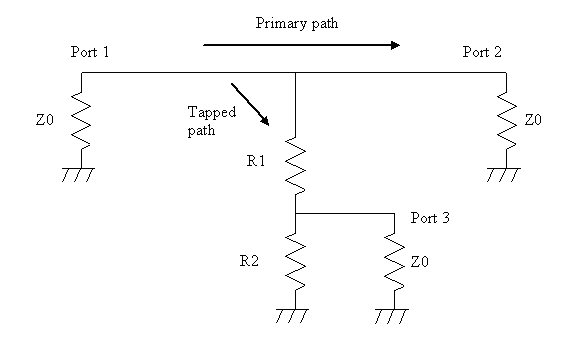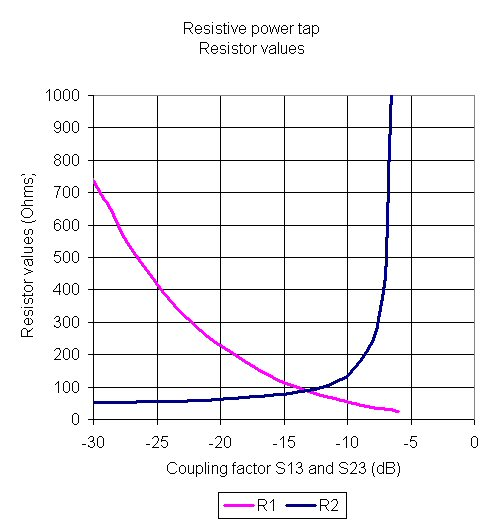# Resistive TapsOh, no! This particular power tap is unmatched, resistive, and has zero directivity, why would you want to use that?

Fear not, Mr. Bill, we haven't seen Sluggo around in ages. But on this page we'll show you that the resistive tap is an excellent technique for coupling power, and often has advantages over all other techniques. The resistive power tap is the answer to the question "how can I create an extremely compact -20 dB coupling network with less than 1 dB loss over infinite bandwidth?"

Advantages of the resistive tap include ultra-wide bandwidth, low insertion loss for low coupling values. For a 20 dB coupler, your circuit might have a hard time noticing the loss in the primary path due to the missing power that is coupled off!

Warning: we must issue the following warning about resistive taps. The accuracy of the coupling coefficient is dependent on your ability to maintain Z0 at ports 1 and 2 in your system. If you were to add a resistive tap to the output of a single-ended power amplifier which might have -6 dB small signal S22, don't bet your paycheck on maintaining that -20 dB coupling coefficient. Recall the maximum power transfer theorem, and you will realize that the coupling has nowhere to go but down, when the impedance matches that the ports see are non-ideal. Your 20 dB coupler could very easily act like a 22 dB coupler. And if the output port opens (failed bond wire?), all bets are off.

The resistive power tap schematic is shown below. In this case, we use R1 and R2 to steal power from the primary path, with the stipulation that port 3 sees an impedance matched to Z0. Using Ohm's law and some manipulation, R1 can be expressed in terms of R2 and Z0 as:

R1=(Z02+Z0R2)/(2R2-2Z0)

The expression for R2 in terms of the voltage coupling factor "CF" from port 1 to port 3 is simply:

R2=Z0/(1-2xCF)

Thus for a -20 dB, 50 ohm coupler, R1 is 225 ohms and R2 is 62.5 ohms.

We'll post expressions for insertion loss and return loss someday. For the above -20 dB example, the insertion loss will be -0.82 dB, if both source and load are perfectly matched to Z0. The match at Ports 1 and 2 are -20.9 dB.A common mistake that engineers make is to create a power tap using just R1. Sure, this brings power to port three, but port 3 will also see a very poor impedance match. Using a combination of R1 and R2, we can match port 3 to Z0 in all cases. This puts a lower limit of 25 ohms on R1 (for R2=infinite), at a maximum coupling of -6.02 dB from port 1 to port 3. At the other extreme, R1 becomes infinite and R2 is 50 ohms when the coupler provides perfect isolation of port 3.Here's a plot of the insertion loss versus coupling factors. By symmetry, S13=S23, and S11=S22. Not plotted is S33, because it's a perfect match to Z0.More to come!

Author : Unknown Editor